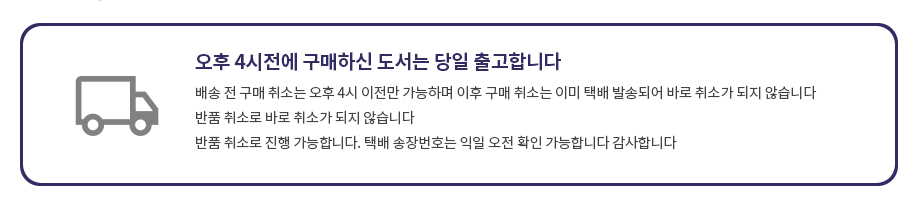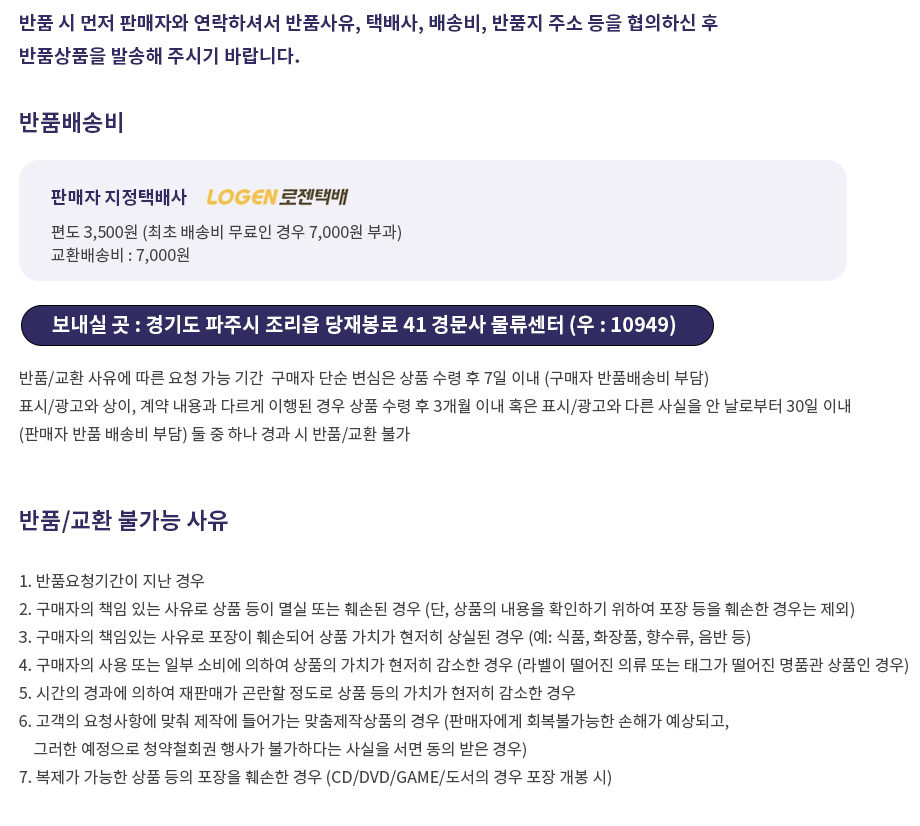## 로그인이필요합니다## 도서를 검색해 주세요.

원하시는 결과가 없으시면 문의 주시거나 다른 검색어를 입력해보세요.

•상품상세

## Probability: An Introduction(1960) 요약정보 및 구매

상품 선택옵션 0 개, 추가옵션 0 개

사용후기 0 개
지은이 Samuel Goldberg 1987-01-01 1판 322 9780486652528 구매가능 14,000원 0점 주문시 결제

### 선택된 옵션

• Probability: An Introduction(1960)
+0원

## 관련상품

• Excellent basic text covers set theory, probability theory for finite sample spaces, binomial theorem, probability distributions, means, standard deviations, probability function of binomial distribution, and other key concepts and methods essential to a thorough understanding of probability. Designed for use by math or statistics departments offering a first course in probability. 360 illustrative problems with answers for half. Only high school algebra needed. Chapter bibliographies.

• 학습자료

등록된 학습자료가 없습니다.

정오표

등록된 정오표가 없습니다.

사용후기가 없습니다.

상품문의가 없습니다.

• ## 배송/교환정보

### 배송정보### 교환/반품 정보### 선택된 옵션

• Probability: An Introduction(1960)
+0원